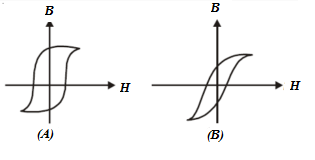# Magnetism MCQs for NEET

The property of any object by virtue of which it attracts or repels magnetic objects is known as magnetism. Magnets can be of two types as Natural magnets and Artificial Magnets. Magnetite and loadstone are examples of natural magnets while a bar magnet, electromagnet and a horseshoe magnet are examples of artificial magnets.

Following are the properties of magnets:

• Like magnetic poles repel each other while unlike magnetic poles attract each other.
• A freely suspended magnet aligns itself into a north-south direction
• Magnetic poles exist in pairs

When a magnetic dipole is placed in a uniform magnetic field then a torque acts on it which is given by the formula:

 $$\tau=MB\sin \theta$$

1. For which of the following is magnetic susceptibility negative?

1. Paramagnetic and Ferromagnetic materials
2. Paramagnetic Materials only
3. Ferromagnetic Materials only
4. Diamagnetic Materials

2. The Hysteresis loops for two magnetic materials A and B are given below:These materials are used to make magnets for transformer core, electric generators and electromagnet core. Which of the following is true about the given magnets?

1. A for transformers and B for electric generators
2. A for electric generators and transformers
3. B for electromagnets and generators
4. A for electromagnets and B for electric generators

Answer: (c) B for electromagnets and generators

3. Which of the following is the unit of magnetic flux density?

1. Weber/meter2
2. Tesla
3. Newton/ampere-metre
4. All of the above

4. The magnetism of a magnet is due to

1. earth
2. cosmic rays
3. due to pressure of big magnet inside the earth
4. spin motion of electrons

Answer: (d) spin motion of electrons

5. The magnetic field at a point x on the axis of a mall bar magnet is equal to the field at a point y on the equator of the same magnet. The ratio of the distances of x and y from the centre of the magnet is

1. 2-1/3
2. 21/3
3. 23
4. 2-3

6. Which of the following materials is the most suitable for making a permanent magnet?

1. Soft Iron
2. Nickel
3. Copper
4. Steel

7. A sensitive magnetic field instrument can be effectively shielded from the external magnetic field by placing it inside which of the following materials?

1. Plastic Material
2. Teak Wood
3. Soft Iron of high permeability
4. A metal of high conductivity

Answer: (c) Soft Iron of high permeability

8. A long magnet is cut into two parts such that the ratio of their lengths is 2:1. What is the ratio pole strength of both the section?

1. 1:2
2. 2:1
3. 4:1
4. Equal

9. Which of the following statements is true about magnetic field intensity?

1. Magnetic field intensity is the number of lines of force crossing per unit volume.
2. Magnetic field intensity is the number of lines of force crossing per unit area.
3. Magnetic field intensity is the magnetic induction force acting on a unit magnetic pole.
4. Magnetic field intensity is the magnetic moment per unit volume.

Answer: (c) Magnetic field intensity is the magnetic induction force acting on a unit magnetic pole

10. What happens to the magnetic needle kept in a non-uniform magnetic field?

1. It experiences force but not torque
2. It experiences torque but not force
3. It experiences both force and torque
4. It neither experiences force nor torque

Answer: (a) It experiences force but not torque

11. What is the torque acting on the bar magnet of moment M which is placed in a uniform field H making an angle of 300 with the field?

1. MH
2. MH/2
3. MH/3
4. MH/4

12. What happens to the magnetic moment if a hole is made at the centre of a bar magnet?

1. Decreases
2. Increases
3. Not a change
4. None of the above

13. Which of the following statements is true about magnetic lines of force?

1. Magnetic lines of force are always closed.
2. Magnetic lines of force always intersect each other.
3. Magnetic lines of force tend to crowd far away from the poles of the magnet
4. Magnetic lines of force do not pass through the vacuum.

Answer: (a) Magnetic lines of force are always closed.

14. What is the work done by the magnet of moment M if it is rotated through 3600 in magnetic field H?

1. 2MH
2. MH
3. 2πMH
4. Zero

15. Magnetic lines of force of a bar magnet do not intersect because

1. The lines have similar charges hence they repel each other
2. The lines always diverge from a single point
3. A point always has a single net magnetic field
4. The lines need magnetic lenses to intersect

Answer: (c) A point always has a single net magnetic field

Stay tuned to BYJU’S and Keep Falling in Love with Learning!

NEET Physics MCQs you cannot miss:

1. Omkar raut

Nice